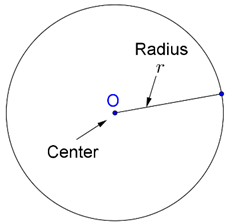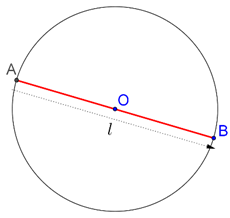# What is a Circle

Go back to  'Circles'

A circle is one of the most perfect figures in geometry. It is also one of the simplest shapes in geometry, and is formed by the set of all points which are at constant distance (radius) from a fixed point (center) in the plane:In the figure above, O is the center and r is the radius of the circle. Any point on the circle will at a distance of r from the center O.

Any circle will divide the plane into: the interior of the circle, and the exterior of the circle. Suppose that the center of a circle is O, and its radius is r. Consider any point P in the plane.

• If P lies in the interior of the circle, then $$OP{\rm{ }} < r$$
• If P lies exactly on the circle, then $$OP{\rm{ }} = r$$
• If P lies in the exterior region of the circle, then $$OP{\rm{ }} > r$$

We define two additional terms related to any circle:

• The diameter of a circle is a length equal to twice its radius. The word diameter can be used interchangeably – it could mean a segment, or it could mean a length. Consider the following figure:We could say that the diameter of this circle is l units, or we could also say that AB is a diameter of this circle whose length is l units.

• The length of the complete arc of a circle is called its circumference.

Learn from the best math teachers and top your exams

• Live one on one classroom and doubt clearing
• Practice worksheets in and after class for conceptual clarity
• Personalized curriculum to keep up with school

0